# US8320048B2 - Projection lens and projection-type display apparatus using the lens - Google Patents

## Info

Publication number
US8320048B2
US8320048B2 US13/079,545 US201113079545A US8320048B2 US 8320048 B2 US8320048 B2 US 8320048B2 US 201113079545 A US201113079545 A US 201113079545A US 8320048 B2 US8320048 B2 US 8320048B2
Authority
US
United States
Prior art keywords
lens
projection
projection lens
formula
light
Prior art date
Legal status (The legal status is an assumption and is not a legal conclusion. Google has not performed a legal analysis and makes no representation as to the accuracy of the status listed.)
Active, expires
Application number
US13/079,545
Other versions
US20110242682A1 (en
Inventor
Chikara Yamamoto
Current Assignee (The listed assignees may be inaccurate. Google has not performed a legal analysis and makes no representation or warranty as to the accuracy of the list.)
Fujifilm Corp
Original Assignee
Fujifilm Corp
Priority date (The priority date is an assumption and is not a legal conclusion. Google has not performed a legal analysis and makes no representation as to the accuracy of the date listed.)
Filing date
Publication date
Priority to JP2010-086578 priority Critical
Priority to JP2010086578A priority patent/JP5468966B2/en
Application filed by Fujifilm Corp filed Critical Fujifilm Corp
Assigned to FUJIFILM CORPORATION reassignment FUJIFILM CORPORATION ASSIGNMENT OF ASSIGNORS INTEREST (SEE DOCUMENT FOR DETAILS). Assignors: YAMAMOTO, CHIKARA
Publication of US20110242682A1 publication Critical patent/US20110242682A1/en
Application granted granted Critical
Publication of US8320048B2 publication Critical patent/US8320048B2/en
Active legal-status Critical Current

• 230000003287 optical Effects 0.000 claims description 34
• 238000005286 illumination Methods 0.000 claims description 18
• 230000000051 modifying Effects 0.000 claims description 9
• 239000003570 air Substances 0.000 claims 10
• 238000010586 diagram Methods 0.000 description 53
• 230000004075 alteration Effects 0.000 description 38
• 239000004973 liquid crystal related substance Substances 0.000 description 17
• 239000003086 colorant Substances 0.000 description 13
• 230000000875 corresponding Effects 0.000 description 13
• 230000004048 modification Effects 0.000 description 9
• 238000006011 modification reaction Methods 0.000 description 9
• 238000000926 separation method Methods 0.000 description 8
• 201000009310 astigmatism Diseases 0.000 description 7
• 230000005499 meniscus Effects 0.000 description 5
• 230000000694 effects Effects 0.000 description 3
• 238000003780 insertion Methods 0.000 description 2
• 229910052710 silicon Inorganic materials 0.000 description 2
• 239000010703 silicon Substances 0.000 description 2

## Images

•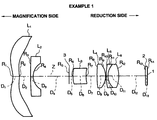•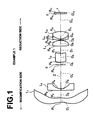•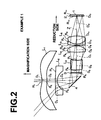•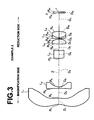•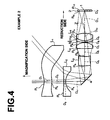•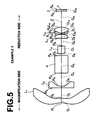•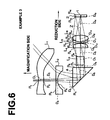•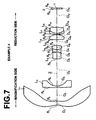•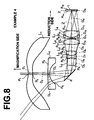•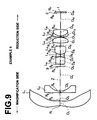•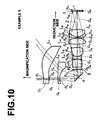•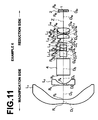•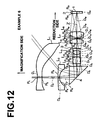•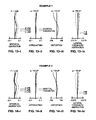•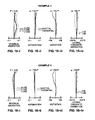•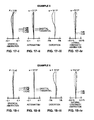•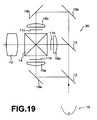•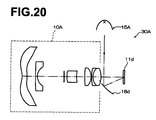## Classifications

• GPHYSICS
• G02OPTICS
• G02BOPTICAL ELEMENTS, SYSTEMS, OR APPARATUS
• G02B13/00Optical objectives specially designed for the purposes specified below
• G02B13/04Reversed telephoto objectives
• GPHYSICS
• G02OPTICS
• G02BOPTICAL ELEMENTS, SYSTEMS, OR APPARATUS
• G02B13/00Optical objectives specially designed for the purposes specified below
• G02B13/22Telecentric objectives or lens systems
• GPHYSICS
• G02OPTICS
• G02BOPTICAL ELEMENTS, SYSTEMS, OR APPARATUS
• G02B7/00Mountings, adjusting means, or light-tight connections, for optical elements
• G02B7/008Mountings, adjusting means, or light-tight connections, for optical elements with means for compensating for changes in temperature or for controlling the temperature; thermal stabilisation

## Abstract

A projection lens includes a first lens having at least an aspheric surface, a second lens having a concave surface facing the reduction side of the projection lens, and having negative refractive power, a third lens, a fourth lens, a fifth lens, a sixth lens having a convex surface facing the reduction side, and having positive refractive power, and the like, which are sequentially arranged from the magnification side of the projection lens. Further, the following formulas (1) and (2) are satisfied:
3.5<Bf/f<7.5  (1); and
1.2<|fa/f|<2.0  (2), where
• f: focal length of the entire system of the projection lens,
• Bf: back focus in air of the entire system, and
• fa: combined focal length of lenses from the second lens to a most-reduction-side lens.

## Description

BACKGROUND OF THE INVENTION
1. Field of the Invention
The present invention relates to a projection lens for magnifying and projecting display information or the like output from a light valve, such as a liquid crystal display device and a DMD (digital micromirror device). Further, the present invention relates to a projection-type display apparatus using the projection lens.
2. Description of the Related Art
In recent years, projection-type display apparatuses using light valves, such as a transmissive or reflective liquid crystal display device and a DMD display device, became widely used. Especially, projection-type display apparatuses using three light valves corresponding to illumination light of RGB primary colors are widely used. In the projection-type display apparatuses using three light valves, rays of illumination light of RGB primary colors are modulated by respective light valves, and combined by a prism or the like. Further, an image is projected onto a screen through a projection lens.
As described above, a prism or the like for combining colors is arranged in the projection-type display apparatus, in which rays of light modulated by three light valves are combined by a color combination optical system. Therefore, a projection lens mounted on the projection-type display apparatus needs to have a long back focus. The long back focus is necessary also to prevent a thermal problem in the apparatus.
Further, in such a projection lens, projection of a large image with a short projection distance is requested. Further, since reduction in the thickness of an apparatus is strongly requested especially in a rear-type projection apparatus, a wide-angle projection lens is requested.
To satisfy such requests, the inventor of the present invention has disclosed a projection lens that has a wide angle of more than or equal to approximately 105 degrees and excellent projection performance, while maintaining a long back focus (please refer to U.S. Pat. No. 7,529,033 (Patent Document 1)).
However, the number of lenses in the projection lens disclosed in Patent Document 1 is in the range of 13 to 16. Therefore, in Patent Document 1, the size and the cost of the projection lens are not reduced in an excellent manner.
Further, reduction in the diameter of a magnification-side lens in the projection lens is requested to reduce the size of the projection lens.
Especially, when a reflective liquid crystal display device or the like is used, a long back focus is requested. However, in the projection lens disclosed in Patent Document 1, the back focus is not always appropriate.
SUMMARY OF THE INVENTION
In view of the foregoing circumstances, it is an object of the present invention to provide a projection lens that has a wide angle and excellent projection performance while maintaining a long back focus, and the size and the cost of which are reducible. Further, it is another object of the present invention to provide a projection-type display apparatus.
A projection lens according to a first aspect of the present invention (corresponding to Claim 1) is a projection lens comprising:
a first lens having at least an aspheric surface;
a second lens having a concave surface facing the reduction side of the projection lens, and having negative refractive power;
a third lens;
a fourth lens having a convex surface facing the reduction side, and having positive refractive power;
a fifth lens having a concave surface facing the reduction side, and having negative refractive power; and
a sixth lens having a convex surface facing the reduction side, and having positive refractive power, which are sequentially arranged from the magnification side of the projection lens,
wherein the following formulas (1) and (2) are satisfied:
3.5<Bf/f<7.5  (1); and
1.2<|fa/f|<2.0  (2), where
f: focal length of the entire system of the projection lens,
Bf: back focus in air of the entire system, and
fa: combined focal length of lenses from the second lens to a most-reduction-side lens (a lens located on the most reduction side among lenses of the projection lens).
A projection lens according to a second aspect of the present invention (corresponding to Claim 2) is a projection lens comprising:
a first lens having at least an aspheric surface;
a second lens having a concave surface facing the reduction side of the projection lens, and having negative refractive power;
a third lens having positive refractive power;
a fourth lens having negative refractive power;
a fifth lens having positive refractive power;
a sixth lens having a convex surface facing the reduction side, and having positive refractive power;
a seventh lens having a concave surface facing the reduction side, and having negative refractive power; and
an eighth lens having a convex surface facing the reduction side, and having positive refractive power, which are sequentially arranged from the magnification side of the projection lens,
wherein the following formulas (1) and (2) are satisfied:
3.5<Bf/f<7.5  (1); and
1.2<|fa/f|<2.0  (2), where
f: focal length of the entire system of the projection lens,
Bf: back focus in air of the entire system, and
fa: combined focal length of lenses from the second lens to a most-reduction-side lens.
In the projection lens according to the first aspect of the present invention and the projection lens according to the second aspect of the present invention, it is desirable that the following formula (3) is satisfied:
3.0<fb/f<6.5  (3), where
fb: combined focal length of three lenses from the reduction side.
In the projection lens according to the first aspect of the present invention and the projection lens according to the second aspect of the present invention, it is desirable that an air space between the second lens and the third lens is longest among air spaces in the projection lens, and that the following formula (4) is satisfied:
5.0<d/f<10.0  (4), where
d: air space between the reduction-side surface of the second lens and the magnification-side surface of the third lens.
In the projection lens according to the first aspect of the present invention and the projection lens according to the second aspect of the present invention, it is desirable that the following formula (5) is satisfied:
ν3<40  (5), where
ν3: Abbe number of the third lens for d-line.
In the projection lens according to the first aspect of the present invention and the projection lens according to the second aspect of the present invention, it is desirable that at least a surface of the third lens is aspheric.
In the projection lens according to the first aspect of the present invention and the projection lens according to the second aspect of the present invention, it is desirable that the following formula (6) is satisfied:
8.0<|f 1 /f|  (6), where
f1: focal length of the first lens.
In the projection lens according to the first aspect of the present invention and the projection lens according to the second aspect of the present invention, it is desirable that the following formula (7) is satisfied:
10.0<|f 3 /f|  (7), where
f3: focal length of the third lens.
In the projection lens according to the first aspect of the present invention and the projection lens according to the second aspect of the present invention, it is desirable that an air space between the second lens and the third lens is longest among air spaces in the projection lens, and that a light deflection means is inserted into the air space between the second lens and the third lens to deflect light.
In the projection lens according to the first aspect of the present invention and the projection lens according to the second aspect of the present invention, it is desirable that the first lens has a non-circular shape including an effective rays passing area.
Further, a projection-type display apparatus according to the present invention is a projection-type display apparatus comprising:
a light source;
a light valve;
an illumination optical unit that guides rays output from the light source to the light valve; and
a projection lens according to the present invention,
wherein the rays output from the light source are optically modulated by the light valve and projected onto a screen by the projection lens.
In the projection lens and the projection-type display apparatus according to the present invention, the projection lens is composed of six or eight lenses, and structured as described above. Therefore, the projection lens has a wide angle and an excellent projection performance, while maintaining a long back focus. Further, it is possible to reduce the size and the cost of the projection lens and the projection-type display apparatus.
When the projection lens satisfies the formula (1), it is possible to prevent the size of the lens system from becoming large, while maintaining a necessary back focus for combining colors and for separating illumination light and projection light from each other.
Further, when the formula (2) is satisfied, it is possible to correct aberration of the most-magnification-side lens (a lens located on the most magnification side among lenses of the projection lens) in an excellent manner, while reducing the size of at least one magnification-side lens in the projection lens.
BRIEF DESCRIPTION OF THE DRAWINGS
FIG. 1 is a diagram illustrating the structure of a projection lens in Example 1 of the present invention;
FIG. 2 is a diagram illustrating the structure of a projection lens in which a mirror for deflecting light is arranged in the projection lens illustrated in FIG. 1;
FIG. 3 is a diagram illustrating the structure of a projection lens in Example 2 of the present invention;
FIG. 4 is a diagram illustrating the structure of a projection lens in which a mirror for deflecting light is arranged in the projection lens illustrated in FIG. 3;
FIG. 5 is a diagram illustrating the structure of a projection lens in Example 3;
FIG. 6 is a diagram illustrating the structure of a projection lens in which the shape of the first lens is changed in the projection lens illustrated in FIG. 5;
FIG. 7 is a diagram illustrating the structure of a projection lens in Example 4;
FIG. 8 is a diagram illustrating the structure of a projection lens in which a mirror for deflecting light is arranged in the projection lens illustrated in FIG. 7;
FIG. 9 is a diagram illustrating the structure of a projection lens in Example 5;
FIG. 10 is a diagram illustrating the structure of a projection lens in which a mirror for deflecting light is arranged and the shape of the first lens and the shape of the second lens are changed in the projection lens illustrated in FIG. 9;
FIG. 11 is a diagram illustrating the structure of a projection lens in Example 6;
FIG. 12 is a diagram illustrating the structure of a projection lens in which a prism for deflecting light is arranged and the shape of the first lens is changed in the projection lens illustrated in FIG. 11;
FIG. 13-i is a diagram illustrating the spherical aberration of the projection lens in Example 1;
FIG. 13-ii is a diagram illustrating the astigmatism of the projection lens in Example 1;
FIG. 13-iii is a diagram illustrating the distortion of the projection lens in Example 1;
FIG. 13-iv is a diagram illustrating the lateral chromatic aberration of the projection lens in Example 1;
FIG. 14-i is a diagram illustrating the spherical aberration of the projection lens in Example 2;
FIG. 14-ii is a diagram illustrating the astigmatism of the projection lens in Example 2;
FIG. 14-iii is a diagram illustrating the distortion of the projection lens in Example 2;
FIG. 14-iv is a diagram illustrating the lateral chromatic aberration of the projection lens in Example 2;
FIG. 15-i is a diagram illustrating the spherical aberration of the projection lens in Example 3;
FIG. 15-ii is a diagram illustrating the astigmatism of the projection lens in Example 3;
FIG. 15-iii is a diagram illustrating the distortion of the projection lens in Example 3;
FIG. 15-iv is a diagram illustrating the lateral chromatic aberration of the projection lens in Example 3;
FIG. 16-i is a diagram illustrating the spherical aberration of the projection lens in Example 4;
FIG. 16-ii is a diagram illustrating the astigmatism of the projection lens in Example 4;
FIG. 16-iii is a diagram illustrating the distortion of the projection lens in Example 4;
FIG. 16-iv is a diagram illustrating the lateral chromatic aberration of the projection lens in Example 4;
FIG. 17-i is a diagram illustrating the spherical aberration of the projection lens in Example 5;
FIG. 17-ii is a diagram illustrating the astigmatism of the projection lens in Example 5;
FIG. 17-iii is a diagram illustrating the distortion of the projection lens in Example 5;
FIG. 17-iv is a diagram illustrating the lateral chromatic aberration of the projection lens in Example 5;
FIG. 18-i is a diagram illustrating the spherical aberration of the projection lens in Example 6;
FIG. 18-ii is a diagram illustrating the astigmatism of the projection lens in Example 6;
FIG. 18-iii is a diagram illustrating the distortion of the projection lens in Example 6;
FIG. 18-iv is a diagram illustrating the lateral chromatic aberration of the projection lens in Example 6;
FIG. 19 is a diagram illustrating the structure of an illumination optical system of a projection-type display apparatus (three panel type); and
FIG. 20 is a diagram illustrating the structure of an illumination optical system of a projection-type display apparatus (single panel type).
DESCRIPTION OF THE PREFERRED EMBODIMENTS
Hereinafter, embodiments of the present invention will be described with reference to drawings. FIG. 1 is a diagram illustrating the structure of a projection lens in Example 1, which will be described later. The first embodiment of the present invention will be described, using the projection lens illustrated in FIG. 1 as an example. FIG. 2 is a diagram illustrating a modification example of the projection lens of Example 1. In the descriptions of the first embodiment, FIG. 2 will also be described.
Further, FIG. 7 is a diagram illustrating the structure of a projection lens in Example 4, which will be described later. The second embodiment of the present invention will be described, using the projection lens illustrated in FIG. 7 as an example. FIG. 8 is a diagram illustrating a modification example of the projection lens of Example 4. In the descriptions of the second embodiment, FIG. 8 will also be described.
In the drawings, line Z represents an optical axis.
<First Embodiment>
A projection lens according to the first embodiment of the present invention is designed to achieve excellent projection performance, while reducing the size of the projection lens (compact projection lens).
As illustrated in FIG. 1, the projection lens according to the first embodiment includes first lens L1 having at least an aspheric surface, second lens L2 having a concave surface facing the reduction side of the projection lens, and having negative refractive power, third lens L3, fourth lens L4 having a convex surface facing the reduction side, and having positive refractive power, fifth lens L5 having a concave surface facing the reduction side, and having negative refractive power, and sixth lens L6 having a convex surface facing the reduction side, and having positive refractive power, which are sequentially arranged from the magnification side of the projection lens. Further, the reduction side of the projection lens is telecentric. Further, a stop (a mask may be provided instead of the stop) 3 may be arranged at a certain position in the lens system (in Example 1, a stop is arranged between the second lens L2 and the third lens L3).
As described above, both surfaces of the first lens L1 may be aspheric. Further, the first lens L1 is formed in such a manner that the refractive power linearly increases, in a positive direction, from the center of the lens toward the periphery of the lens (When the refractive power of the lens on optical axis Z is positive, the lens is formed in such a manner that the positive refractive power increases toward the periphery of the lens. When the refractive power of the lens on optical axis Z is negative, the lens is formed in such a manner that first, the negative refractive power becomes weaker toward the periphery of the lens to reach zero, and after reaching zero, positive refractive power increases toward the periphery of the lens).
Further, it is desirable that the fifth lens L5 and the sixth lens L6 are cemented together to form a cemented lens (Examples 1 and 2, which will be described later), or arranged close to each other (Example 3, which will be described later).
In FIG. 1, rays of light enter an image display plane 1 of a light valve from the right side of the paper, and image information is given to the rays of light at the image display plane 1. Further, the rays of light pass through a filter 2 (various kinds of filter), and enter the projection lens. The rays of light are magnified and projected toward the left side of the paper by the projection lens. In FIG. 1, only one image display plane 1 is illustrated so that the structure is easily recognizable. Alternatively, a projection-type display apparatus may display a full color image by separating rays of light output from a light source into rays of light of three primary colors by a color separation optical system, and by providing three light valves for the rays of light of three primary colors, respectively. When a color combination optical system, such as a cross dichroic prism, is arranged between the image display plane 1 and the sixth lens L6 (when the light valve is a reflective liquid crystal display panel, a polarization plate or a ¼ wavelength plate is used in addition to the light valve), the rays of light of three primary colors can be combined together.
Further, it is desirable that an air space between the second lens L2 and the third lens L3 is longest among air spaces in the projection lens. Further, it is desirable that a light deflection means 4 is inserted into the air space between the second lens L2 and the third lens L3 to deflect the optical path of the projection lens. In the modified example illustrated in FIG. 2, a light reflection mirror, as the light deflection means 4, is arranged between the second lens L2 and the third lens L3 in the projection lens illustrated in FIG. 1 to deflect rays of light only by 90 degrees (in the modification example of Example 2, a light reflection mirror is arranged, and in Example 3 and the modification example of Example 3, a light reflection prism is arranged). Therefore, it is possible to reduce the size of the optical system appropriately based on the size of a space for storing the optical system. When the light deflection means 4 is a light reflection prism, it is possible to reduce the length of the entire system, compared with the case of using a light reflection mirror.
In the modification examples of Examples 2 and 3, which will be described later, the first lens L1 has a non-circular shape including an effective rays passing area, as illustrated in FIGS. 4 and 6. In other words, a part of the first lens L1 is cut, and the first lens L1 is not rotary symmetric.
The first lens L1 is formed in such a manner to prevent the diameter of the first lens L1 from becoming too large. In the present embodiment, the first lens L1 has a non-circular shape including an effective rays passing area, and an unnecessary lens portion is not formed in the first lens L1 (by performing so-called D-cut). Therefore, the size of the first lens L1 is reduced, and the size of the entire lens system is reduced.
In the projection lens of the present embodiment, it is desirable that at least a surface of at least the third lens L3 is aspheric in addition to at least a surface of the first lens L1. Consequently, it is possible to improve the projection performance of the projection lens, while reducing the number of lenses in the projection lens.
In the projection lens of the present embodiment, it is desirable that both of the following formulas (1) and (2) are satisfied:
3.5<Bf/f<7.5  (1); and
1.2<|fa/f|<2.0  (2), where
f: focal length of the entire system of the projection lens,
Bf: back focus in air of the entire system, and
fa: combined focal length of lenses from the second lens to a most-reduction-side lens (sixth lens L6).
The projection lens structured as described above has a wide angle and excellent projection performance, while maintaining a long back focus.
When the formula (1) is satisfied, it is possible to prevent the size of the lens system from becoming large, while maintaining a back focus necessary for color combination processing and light separation processing, which separates illumination light and projection light from each other. Specifically, when the value of Bf/f is lower than the lower limit defined by the formula (1), it becomes difficult to maintain a necessary back focus for color combination processing and light separation processing of illumination light and projection light. When the value of Bf/f exceeds the upper limit defined by the formula (1), the size of the lens system becomes large. When a back focus in a range satisfying the formula (1) is maintained, it is possible to adopt a light valve of a reflective liquid crystal type (LCOS (liquid crystal on silicon) type) in an excellent manner. The light valve of the reflective liquid crystal type requires insertion of a color separation/combination prism, a polarization plate, a ¼ wavelength plate, or the like into the system.
Therefore, it is desirable that the following formula (1′) is satisfied instead of the formula (1). Further, it is more desirable that the following formula (1″) is satisfied instead of the formula (1):
4.0<Bf/f<6.0  (1′); and
4.0<Bf/f<5.0  (1″).
Further, when the formula (2) is satisfied, it is possible to correct aberration of the most-magnification-side lens in an excellent manner, while reducing the size of at least one magnification-side lens. Specifically, when the value of |fa/f| is lower than the lower limit defined by the formula (2), the size of at least one magnification-side lens becomes large. When the value of |fa/f| exceeds the upper limit defined by the formula (2), it becomes difficult to correct aberration of the most-magnification-side lens in an excellent manner.
Therefore, it is desirable that the following formula (2′) is satisfied instead of the formula (2). Further, it is more desirable that the following formula (2″) is satisfied instead of the formula (2):
1.3<|fa/f|<1.8  (2′); and
1.4<|fa/f|<1.7  (2″).
In the projection lens according to the present embodiment, it is desirable that at least one of the following formulas (3) through (7) is satisfied in addition to the formulas (1) and (2):
3.0<fb/f<6.5  (3);
5.0<d/f<10.0  (4);
ν3<40  (5);
8.0<|f 1 /f|  (6); and
10.0<|f 3 /f|  (7), where
fb: combined focal length of three lenses from the reduction side (lenses L4, L5, L6),
d: air space between the reduction-side surface of the second lens L2 and the magnification-side surface of the third lens L3,
ν3: Abbe number of the third lens L3 for d-line,
f1: focal length of the first lens L1, and
f3: focal length of the third lens L3.
The formula (4) is based on the premise that an air space between the second lens L2 and the third lens L3 is longest among air spaces in the projection lens.
Next, the meanings of the formulas (3) through (7) will be described.
When the formula (3) is satisfied, it is possible to prevent the refractive power from becoming too large and to correct various kinds of aberration in an excellent manner. Further, it is possible to prevent aback focus from becoming too long, and to correct lateral chromatic aberration in an excellent manner. Specifically, when the value of fb/f is lower than the lower limit defined by the formula (3), the refractive power is too strong, and it becomes difficult to correct aberration. When the value of fb/f exceeds the upper limit defined by the formula (3), aback focus becomes too long, and lateral chromatic aberration is insufficiently corrected.
Therefore, it is desirable that the following formula (3′) is satisfied instead of the'formula (3). Further, it is more desirable that the following formula (3″) is satisfied instead of the formula (3):
4.0<fb/f<6.0  (3′); and
4.0<fb/f<4.6  (3″).
Further, when the formula (4) is satisfied, it is possible to set an appropriate back focus to arrange a light deflection means between lenses, and to prevent the size of the lens system from becoming large. Specifically, when the value of d/f is lower than the lower limit defined by the formula (4), a back focus becomes too short, and it becomes difficult to arrange the light deflection means 4 between lenses. When the value of d/f exceeds the upper limit defined by the formula (4), the size of the lens system becomes large.
Therefore, it is desirable that the following formula (4′) is satisfied instead of the formula (4). Further, it is more desirable that the following formula (4″) is satisfied instead of the formula (4):
5.5<d/f<9.0  (4′); and
6.0<d/f<8.5  (4″).
When the formula (5) is satisfied, it is possible to correct longitudinal chromatic aberration in an excellent manner. Specifically, when the value of ν3 is lower than the lower limit defined by the formula (5), it becomes difficult to correct longitudinal chromatic aberration in an excellent manner.
Therefore, it is desirable that the following formula (5′) is satisfied instead of the formula (5):
ν3<30  (5′).
Further, when at least one of the formulas (6) and (7) is satisfied, it is possible to suppress deterioration in performance caused by a change in temperature. Specifically, when the value of |f1/f| is lower than the lower limit defined by the formula (6), and the value of |f3/f| is lower than the lower limit defined by the formula (7), deterioration in performance by a change in temperature becomes intolerable.
Therefore, it is desirable that the following formulas (6′) and (7′) are satisfied instead of the formulas (6) and (7). Further, it is more desirable that the following formula (6″) is satisfied instead of the formula (6):
8.0<|f 1 /f|<20.0  (6′);
10.0<|f 1 /f|<16.0  (6″); and
20.0<|f 3 /f|<30.0  (7′).
<Second Embodiment>
A projection lens according to the second embodiment is designed in such a manner to have excellent projection performance, while reducing the size of the projection lens.
Specifically, as illustrated in FIG. 7, the projection lens according to the second embodiment includes first lens L1 having at least an aspheric surface, second lens L2 having a concave surface facing the reduction side of the projection lens, and having negative refractive power, third lens L3 having positive refractive power, fourth lens L4 having negative refractive power, fifth lens L5 having positive refractive power, sixth lens L6 having a convex surface facing the reduction side, and having positive refractive power, seventh lens L7 having a concave surface facing the reduction side, and having negative refractive power, and eighth lens L8 having a convex surface facing the reduction side, and having positive refractive power, which are sequentially arranged from the magnification side of the projection lens. Further, the reduction side of the projection lens is substantially telecentric. Further, a stop (a mask may be provided instead of the stop) 3 may be arranged at a certain position in the lens system (in Example 5, a stop is arranged between the second lens L2 and the third lens L3, and in Example 6, a stop is arranged between the fifth lens L5 and the sixth lens L6).
As described above, both surfaces of the first lens L1 may be aspheric. Further, the first lens L1 is formed in such a manner that the refractive power linearly increases, in a positive direction, from the center of the lens toward the periphery of the lens (When the refractive power of the lens on optical axis Z is positive, the lens is formed in such a manner that the positive refractive power increases toward the periphery of the lens. When the refractive power of the lens on optical axis Z is negative, the lens is formed in such a manner that first, the negative refractive power becomes weaker toward the periphery of the lens to reach zero, and after reaching zero, positive refractive power increases toward the periphery of the lens).
Further, it is desirable that the fourth lens L4 and the fifth lens L5 are cemented together to form a cemented lens, and the seventh lens L7 and the eighth lens L8 are cemented together to form a cemented lens (Examples 4 and 5, which will be described later), or the fourth lens L4 and the fifth lens L5 are arranged close to each other, and the seventh lens L7 and the eighth lens L8 are arranged close to each other (Example 6, which will be described later).
In FIG. 7, rays of light enter an image display plane 1 of a light valve from the right side of the paper, and image information is given to the rays of light at the image display plane 1. Further, the rays of light pass through a filter 2 (various kinds of filter), and enter the projection lens. Further, the rays of light are magnified and projected toward the left side of the paper by the projection lens. In FIG. 7, only one image display plane 1 is illustrated so that the structure is easily recognizable. Alternatively, a projection-type display apparatus may display a full color image by separating rays of light output from a light source into rays of light of three primary colors by a color separation optical system, and by providing three light valves for the rays of light of three primary colors, respectively.
When a color combination optical system, such as a cross dichroic prism, is arranged between the image display plane 1 and the eighth lens L8 (when the light valve is a reflective liquid crystal display panel, a polarization plate or a ¼ wavelength plate is used in addition to the light valve), the rays of light of three primary colors can be combined together.
Further, it is desirable that an air space between the second lens L2 and the third lens L3 is longest among air spaces in the projection lens. Further, it is desirable that a light deflection means 4 is inserted into the air space between the second lens L2 and the third lens L3 to deflect the optical path of the projection lens. In the modified example illustrated in FIG. 8, a light reflection mirror, as the light deflection means 4, is arranged between the second lens L2 and the third lens L3 in the projection lens illustrated in FIG. 7 to deflect light only by 90 degrees (in the modification examples of Examples 4 and 5, a light reflection mirror is arranged, and in Example 6 and the modification example of Example 6, a light reflection prism is arranged). Therefore, it is possible to reduce the size of the optical system appropriately based on the size of a space for storing the optical system. When the light deflection means 4 is a light reflection prism, it is possible to reduce the entire length, compared with the case of using a light reflection mirror.
In the modification examples of Example 5, which will be described later, each of the first lens L1 and the second lens L2 has a non-circular shape including an effective rays passing area, as illustrated in FIG. 10. In other words, a part of the first lens L1 and a part of the second lens L2 are cut, and the first lens L1 and the second lens L2 are not rotary symmetric. Further, in the modified example of Example 6, the first lens L1 has a non-circular shape including an effective rays passing area, as illustrated in FIG. 12. In other words, a part of the first lens L1 is cut, and the first lens L1 is not rotary symmetric.
The first lens L1 (and the second lens L2) is formed as described above to prevent the diameter of the first lens L1 (and the second lens L2) from becoming too large. In the present embodiment, the first lens L1 (and the second lens L2) has a non-circular shape including an effective rays passing area, and an unnecessary lens portion is not formed (by performing so-called D-cut). Therefore, the size of the first lens L1 (and the second lens L2) is reduced, and the size of the entire lens system is reduced.
In the projection lens of the present embodiment, it is desirable that at least a surface of the first lens L1 is aspheric. Consequently, it is possible to improve the projection performance of the projection lens, while reducing the number of lenses in the projection lens.
In the projection lens of the present embodiment, it is desirable that both of the following formulas (1) and (2) are satisfied:
3.5<Bf/f<7.5  (1); and
1.2<|fa/f|<2.0  (2), where
f: focal length of the entire system of the projection lens,
Bf: back focus in air of the entire system, and
fa: combined focal length of lenses from the second lens to a most-reduction-side lens (eighth lens L8).
When the projection lens is structured as described above, the projection lens has a wide angle and excellent projection performance, while maintaining a long back focus.
When the formula (1) is satisfied, it is possible to prevent the size of the lens system from becoming large, while maintaining a back focus necessary for color combination processing and light separation processing, in which illumination light and projection light are separated from each other. Specifically, when the value of Bf/f is lower than the lower limit defined by the formula (1), it becomes difficult to maintain a necessary back focus for color combination processing and light separation processing of illumination light and projection light. When the value of Bf/f exceeds the upper limit defined by the formula (1), the size of the lens system becomes large. When a back focus in a range satisfying the formula (1) is maintained, it is possible to adopt a light valve of a reflective liquid crystal type (LCOS (liquid crystal on silicon) type) in an excellent manner. The light valve of the reflective liquid crystal type requires insertion of a color separation/combination prism, a polarization plate, a ¼ wavelength plate, or the like into the system.
Therefore, it is desirable that the following formula (1′) is satisfied instead of the formula (1). Further, it is more desirable that the following formula (1′″) is satisfied instead of the formula (1):
4.0<Bf/f<6.0  (1′); and
4.0<Bf/f<5.0  (1′″).
Further, when the formula (2) is satisfied, it is possible to correct aberration of the most-magnification-side lens in an excellent manner, while reducing the size of at least one magnification-side lens. Specifically, when the value of |fa/f| is lower than the lower limit defined by the formula (2), the size of at least one magnification-side lens becomes large. When the value of |fa/f| exceeds the upper limit defined by the formula (2), it becomes difficult to correct aberration of the most-magnification-side lens in an excellent manner.
Therefore, it is desirable that the following formula (2′) is satisfied instead of the formula (2). Further, it is more desirable that the following formula (2″) is satisfied instead of the formula (2)
1.3<|fa/f|<1.8  (2′); and
1.4<|fa/f|<1.7  (2″).
In the projection lens according to the present embodiment, it is desirable that at least one of the following formulas (3), (4) and (6) is satisfied in addition to the formulas (1) and (2):
3.0<fb/f<6.5  (3);
5.0<d/f<10.0  (4); and
8.0<|f 1 /f|  (6), where
fb: combined focal length of three lenses from the reduction side (lenses L6, L7, L8),
d: air space between the reduction-side surface of the second lens L2 and the magnification-side surface of the third lens L3, and
f1: focal length of the first lens L1.
The formula (4) is based on the premise that an air space between, the second lens L2 and the third lens L3 is longest among air spaces in the projection lens.
Next, the meanings of the formulas (3), (4) and (6) will be described.
When the formula (3) is satisfied, it is possible to prevent the refractive power from becoming too large and to correct various kinds of aberration in an excellent manner. Further, it is possible to prevent aback focus from becoming too long, and to correct lateral chromatic aberration in an excellent manner. Specifically, when the value of fb/f is lower than the lower limit defined by the formula (3), the refractive power is too strong, and it becomes difficult to correct aberration. When the value of fb/f exceeds the upper limit defined by the formula (3), a back focus becomes too long, and lateral chromatic aberration is insufficiently corrected.
Therefore, it is desirable that the following formula (3′) is satisfied instead of the formula (3). Further, it is more desirable that the following formula (3′″) is satisfied instead of the formula (3):
4.0<fb/f<6.0  (3′); and
4.6<fb/f<4.6  (3′″)
Further, when the formula (4) is satisfied, it is possible to set an appropriate back focus to arrange a light deflection means between lenses, and to prevent the size of the lens system from becoming large. Specifically, when the value of d/f is lower than the lower limit defined by the formula (4), a back focus becomes too short, and it becomes difficult to arrange the light deflection means 4 between lenses. When the value of d/f exceeds the upper limit defined by the formula (4), the size of the lens system becomes large.
Therefore, it is desirable that the following formula (4′) is satisfied instead of the formula (4). Further, it is more desirable that the following formula (4″) is satisfied instead of the formula (4):
5.5<d/f<9.0  (4′); and
6.0<d/f<8.5  (4″).
When the formula (6) is satisfied, it is possible to suppress deterioration in performance caused by a change in temperature. Specifically, when the value of |f1/f| is lower than the lower limit defined by the formula (6), deterioration in performance caused by a change in temperature becomes intolerable.
Therefore, it is desirable that the following formula (6′) is satisfied instead of the formula (6). Further, it is more desirable that the following formula (6″) is satisfied instead of the formula (6):
8.0<|f 1 /f|<20.0  (6′); and
10.0<|f 1 /f|<16.0  (6″).
Next, a projection-type display device according to an embodiment of the present invention will be described.
FIG. 19 is a diagram illustrating the structure of an illumination optical system 30 and a projection lens 10, which are a main part of a projection-type display apparatus of three panel type.
As illustrated in FIG. 19, the illumination optical system 30 includes a light source 15, transmissive liquid crystal panels 11 a through 11 c, as light valves, dichroic mirrors 12, 13 for separating colors, a cross dichroic prism 14 for combining colors, condenser lenses 16 a through 16 c, and total reflection mirrors 18 a through 18 c. The light source 15 is arranged before the dichroic mirror 12. White light output from the light source 15 enters, through an illumination optical system, liquid crystal panels 11 a through 11 c corresponding to rays of light of three colors (G light, B light and R light), respectively, and is modulated. Further, the modulated light is projected onto a screen, which is not illustrated, by the projection lens 10.
The projection-type display apparatus of three panel type uses the projection lens of the present invention. Therefore, it is possible to reduce the size and the cost of the projection-type display apparatus, while maintaining a long back focus and achieving a wide angle and excellent projection performance.
FIG. 20 is a diagram illustrating the structure of an illumination optical system 30A and a projection lens 10A, which are a main part of a projection-type display apparatus of single panel type.
As illustrated in FIG. 20, the illumination optical system 30A includes a light source 15A for outputting white light, a reflective liquid crystal panel 11 d, as a light valve, and a total reflection mirror 18 d, which has a concave surface. Rays of light from the light source 15A are reflected and converged toward the direction of the reflective liquid crystal panel 11 d by the total reflection mirror 18 d. The white light output from the light source 15A enters, through the total reflection mirror 18 d, the liquid crystal panel lid, and is optically modulated. Further, the modulated light is projected onto a screen, which is not illustrated, by the projection lens 10A.
The projection-type display apparatus of single panel type uses the projection lens of the present invention. Therefore, it is possible to reduce the size and the cost of the projection-type display apparatus, while maintaining a long back focus. Further, a wide angle and excellent projection performance are achieved.
Next, specific examples of the projection lens according to the present invention will be described. In the descriptions of the following examples, the same reference numerals are'assigned to elements that have similar actions and effects.
EXAMPLE 1
As illustrated in FIG. 1, a projection lens in Example 1 includes six lenses L1 through L6, which are sequentially arranged from the magnification side of the projection lens, and an aperture stop (a mask may be provided instead of the aperture stop) 3. Further, the reduction side of the projection lens is substantially telecentric. FIG. 2 is a diagram illustrating a case of arranging a light reflection mirror, as the light deflection means 4 for deflecting light, between the second lens L2 and the third lens L3 in the projection lens illustrated in FIG. 1.
Specifically, first lens L1, second lens L2, an aperture stop (a mask may be provided instead of the aperture stop) 3, third lens L3, fourth lens L4, fifth lens L5, and sixth lens L6 are sequentially arranged from the magnification side of the projection lens. The first lens L1 has small refractive power, and both surfaces of the first lens L1 are aspheric. The second lens L2 is a negative meniscus lens having a concave surface facing the reduction side of the projection lens. The third lens L3 has small refractive power, and both surfaces of the third lens L3 are aspheric. The fourth lens L4 is a double convex lens. The fifth lens L5 is a negative meniscus lens having a concave surface facing the reduction side. The sixth lens L6 is a double convex lens. Further, the fifth lens L5 and the sixth lens L6 are cemented together to form a cemented lens of two lenses.
The shape of each aspheric surface is defined by the following aspheric equation, which represents an aspheric surface. The first lens L1 and the third lens L3, each including at least an aspheric surface, can achieve an advantageous effect of the present invention if at least a surface is aspheric. However, it is more desirable that both surfaces are aspheric.
$Z = Y 2 / R 1 + 1 - K × Y 2 / R 2 + ∑ i = 3 12 ⁢ A i ⁢ Y i , where [ Equation ⁢ ⁢ 1 ]$
Z: length of a perpendicular from a point on an aspheric surface, the point away from optical axis by distance Y, to flat plane (flat plane perpendicular to the optical axis) in contact with the vertex of the aspheric surface,
Y: distance from the optical axis,
R: curvature radius of the aspheric surface in the vicinity of the optical axis,
K: eccentricity, and
Ai: aspheric coefficient (i=3 through 12).
Further, the projection lens in Example 1 satisfies the following formulas (1) through (7), (1′) through (7′), (1″) through (4″), and (6″).
Further, an image display plane 1 of a light valve and a filter 2 (various kinds of filters) are illustrated, in FIG. 1. Ina modified example of the projection lens of Example 1, an air space in which a light reflection mirror 4 for deflecting light is arrangeable is provided between the second lens L2 and the third lens L3 (stop 3), as illustrated in FIG. 2. The projection lens is structured in such a manner that the reduction side is telecentric.
Table 1 shows data about the projection lens in Example 1. Table 1 shows curvature radius R of each lens surface (normalized by assuming the focal length to be 1; same in the following examples), the center thickness D of each lens and air space D between lenses (normalized by assuming the focal length to be 1, same in the following examples) (hereinafter, the center thickness D and the air space D are referred to as “axial surface distance”), and refractive index Nd and Abbe number νd of each lens for d-line. In Table 1, and the following tables, surface numbers start from the magnification side of the projection lens. Further, mark “*” is attached to the right side of the surface number of an aspheric surface. In Example 1, and the following Examples 2 through 6, the tables show curvature radius R of an aspheric surface on optical axis Z. However, in the diagrams illustrating the structure of the lenses corresponding to the examples, a line indicating the curvature radius R is not always drawn from an intersection with optical axis Z to make the diagrams easily recognizable.
Further, Table 2 shows aspheric coefficients K, A3 through A12 for the aspheric surfaces.
 TABLE 1 ABBE SURFACE CURVATURE DISTANCE REFRACTIVE NUMBER NUMBER RADIUS (R) (D) INDEX (Nd) (νd)
 OBJ ∞ 111.85 1 * −2.865 1.05 1.491000 57.6 2 * −6.094 1.80 3 56.155 0.40 1.804000 46.6 4 2.366 5.70 5 (MASK) ∞ 0.62 6 * 5.826 2.07 1.631800 23.2 7 * 7.899 1.74 8 17.845 1.38 1.517400 52.4 9 −2.851 0.10 10 8.993 0.29 1.846700 23.8 11 2.601 1.73 1.487500 70.2 12 −4.620 4.22 13 ∞ 0.31 1.487500 70.2 14 ∞ * ASPHERIC SURFACE
 TABLE 2 ASPHERIC COEFFICIENT
 SURFACE NUMBER K A3 A4 A5 A6 1 0.00000 6.19881E−02 −7.85767E−03 1.31173E−03 −1.98670E−04 2 0.00000 2.34938E−02 1.20264E−02 −2.19444E−03 −1.41495E−04 6 1.00000 0.00000E+00 3.19509E−03 0.00000E+00 1.75280E−02 7 1.00000 0.00000E+00 3.27452E−02 0.00000E+00 2.64792E−03
 SURFACE NUMBER A7 A8 A9 A10 A11 A12 1 4.81179E−06 −3.54502E−06 2.35813E−06 −4.24493E−07 3.30411E−08 −9.75840E−10 2 1.06233E−05 1.92512E−06 8.93649E−07 −1.93273E−07 1.41346E−08 −3.91085E−10 6 0.00000E+00 −1.22542E−02 0.00000E+00 3.02798E−03 0.00000E+00 0.00000E+00 7 0.00000E+00 3.54601E−03 0.00000E+00 −6.94095E−04 0.00000E+00 0.00000E+00
In Example 1, values corresponding to the formulas (1) through (7), (1′) through (7′), (1″) through (4″), and (6″) are shown in Table 13, which will be described later. Example 1 satisfies all of the formulas.
EXAMPLE 2
FIG. 3 illustrates the structure of the projection lens in Example 2. The structure of the projection lens in Example 2 is substantially similar to the projection lens in Example 1. However, Example 2 differs in that the second lens L2 is a double concave lens, and that an aperture stop (mask) 3 is not arranged on optical axis Z. Further, FIG. 4 is a diagram illustrating a modified example of Example 2. In the modified example, a light reflection mirror, as a light deflection means 4 for deflecting light, is arranged between the second lens L2 and the third lens L3 of the projection lens illustrated in FIG. 3.
Table 3 shows data about the projection lens in Example 2. Table 3 shows curvature radius R of each lens surface, the axial surface distance D of each lens, and refractive index Nd and Abbe number νd of each lens for d-line. Further, Table 4 shows aspheric coefficients K, A3 through A12 for the aspheric surfaces.
 TABLE 3 ABBE SURFACE CURVATURE DISTANCE REFRACTIVE NUMBER NUMBER RADIUS (R) (D) INDEX (Nd) (νd)
 OBJ ∞ 113.44 1 * −2.621 1.57 1.491000 57.6 2 * −5.413 2.50 3 −37.638 0.41 1.729200 54.7 4 2.777 7.19 5 * 7.510 1.95 1.631800 23.2 6 * 13.669 1.69 7 44.503 1.25 1.497000 81.5 8 −2.579 0.10 9 8.792 0.29 1.903700 31.3 10 2.667 1.56 1.438800 94.9 11 −4.141 4.61 12 ∞ 0.31 1.487500 70.2 13 ∞ * ASPHERIC SURFACE
 TABLE 4 ASPHERIC COEFFICIENT
 SURFACE NUMBER K A3 A4 A5 A6 1 0.00000 5.20081E−02 −1.87581E−03 −6.65711E−05 −1.44665E−04 2 0.00000 1.90144E−02 1.35013E−02 −1.94540E−03 −1.51362E−04 5 1.00000 0.00000E+00 7.03400E−03 0.00000E+00 1.09608E−02 6 1.00000 0.00000E+00 3.50423E−02 0.00000E+00 −4.10959E−04
 SURFACE NUMBER A7 A8 A9 A10 A11 A12 1 2.44920E−05 −3.42680E−06 1.62289E−06 −3.39722E−07 2.86882E−08 −8.35394E−10 2 5.80872E−06 1.41757E−06 8.03928E−07 −1.62478E−07 1.22725E−08 −3.34799E−10 5 0.00000E+00 −6.68518E−03 0.00000E+00 1.52312E−03 0.00000E+00 0.00000E+00 6 0.00000E+00 6.42895E−03 0.00000E+00 −8.26772E−04 0.00000E+00 0.00000E+00
In Example 2, values corresponding to the formulas (1) through (7), (1′) through (7′), (1″) through (4″), and (6″) are shown in Table 13, which will be described later. Example 2 satisfies all of the formulas.
EXAMPLE 3
FIG. 5 illustrates the structure of the projection lens in Example 3. The structure of the projection lens in Example 3 is substantially similar to the projection lens in Example 2. However, Example 3 differs in that the fourth lens L4 is a positive meniscus lens having a convex surface facing the reduction side, and that a light reflection prism, as a light deflection means 4, is arranged between the second lens L2 and the third lens L, and that the fifth lens L5 and the sixth lens L6 are arranged close to each other with a very small distance therebetween. Since the light reflection prism is used as the light deflection means 4, it is possible to reduce the entire length, compared with the examples illustrated in FIGS. 2 and 4, in which a light reflection mirror is used as the light deflection means 4.
The projection lens illustrated in FIG. 6 is a modified example of the projection lens of Example 3. In the projection lens illustrated in FIG. 6, a light reflection prism, as a light deflection means 4 for deflecting light, is arranged between the second lens L2 and the third lens L3 of the projection lens illustrated in FIG. 5. In the projection lens illustrated in FIG. 6, the first lens L1 has a non-circular shape including an effective rays passing area. Specifically, a part of the first lens L1 is cut, and the first lens L1 is not rotary symmetric. Accordingly, the size of the lens system is greatly reducible.
Table 5 shows data about the projection lens in Example 3. Table 5 shows curvature radius R of each lens surface, the axial surface distance D of each lens, and refractive index Nd and Abbe number νd of each lens for d-line. Further, Table 6 shows aspheric coefficients K, A3 through A12 for the aspheric surfaces.
 TABLE 5 ABBE SURFACE CURVATURE DISTANCE REFRACTIVE NUMBER NUMBER RADIUS (R) (D) INDEX (Nd) (νd)
 OBJ ∞ 112.87 1 * −2.919 1.25 1.491000 57.6 2 * −5.748 2.88 3 −95.171 0.41 1.713000 53.9 4 2.783 3.59 5 ∞ 4.80 1.516300 64.1 6 ∞ 0.62 7 * 12.284 1.98 1.846700 23.8 8 * 30.325 2.15 9 −169.476 1.07 1.497000 81.5 10 −3.481 0.10 11 9.239 0.29 1.846700 23.8 12 3.081 0.01 13 3.076 1.59 1.516300 64.1 14 −4.364 4.37 15 ∞ 0.53 1.487500 70.2 16 ∞ * ASPHERIC SURFACE
 TABLE 6 ASPHERIC COEFFICIENT
 SURFACE NUMBER K A3 A4 A5 A6 1 0.00000 5.84977E−02 −7.39995E−03 1.19496E−03 −1.81294E−04 2 0.00000 2.24349E−02 1.24117E−02 −2.05302E−03 −1.35367E−04 7 1.00000 0.00000E+00 −2.13273E−03 0.00000E+00 2.51331E−02 8 1.00000 0.00000E+00 1.72297E−02 0.00000E+00 −6.23290E−03
 SURFACE NUMBER A7 A8 A9 A10 A11 A12 1 6.14067E−06 −3.50686E−06 2.12260E−06 −3.86485E−07 3.01796E−08 −8.83288E−10 2 9.26551E−06 1.65931E−06 8.12913E−07 −1.79551E−07 1.29104E−08 −3.53993E−10 7 0.00000E+00 −2.62955E−02 0.00000E+00 9.84411E−03 0.00000E+00 0.00000E+00 8 0.00000E+00 1.00535E−02 0.00000E+00 −4.07938E−03 0.00000E+00 0.00000E+00
In Example 3, values corresponding to the formulas (1) through (7), (1′) through (7′), (1″) through (4″), and (6″) are shown in Table 13, which will be described later. Example 3 satisfies all of the formulas.
EXAMPLE 4
As illustrated in FIG. 7, a projection lens in Example 4 includes eight lenses L1 through L8, which are sequentially arranged from the magnification side of the projection lens. Further, the reduction side of the projection lens is substantially telecentric. FIG. 8 is a diagram illustrating a case of arranging a light reflection mirror, as the light deflection means 4 for deflecting light, between the second lens L2 and the third lens L3 in the projection lens illustrated in FIG. 7.
Specifically, first lens L1, second lens L2, third lens L3, fourth lens L4, fifth lens L5, sixth lens L6, seventh lens L7 and eighth lens L8 are sequentially arranged from the magnification side of the projection lens. The first lens L1 has small refractive power, and both surfaces of the first lens L1 are aspheric. The second lens L2 is a negative meniscus lens having a concave surface facing the reduction side of the projection lens. The third lens L3 is a double convex lens. The fourth lens L4 is a double concave lens. The fifth lens L5 is a double convex lens. The sixth lens L6 is a double convex lens. The seventh lens L7 is a negative meniscus lens having a concave surface facing the reduction side. The eighth lens L8 is a double convex lens. Further, the fourth lens L4 and the fifth lens L5 are cemented together to form a cemented lens of two lenses. Further, the seventh lens L7 and the eighth lens L8 are cemented together to form a cemented lens of two lenses.
The shape of each aspheric surface is defined by the aforementioned aspheric equation. The first lens L1, which includes at least an aspheric surface, can achieve an advantageous effect of the present invention if at least a surface is aspheric. However, it is more desirable that both surfaces are aspheric.
Table 7 shows data about the projection lens in Example 4. Table 7 shows curvature radius R of each lens surface, the axial surface distance D of each lens, and refractive index Nd and Abbe number νd of each lens for d-line. Further, Table 8 shows aspheric coefficients K, A3 through A12 for the aspheric surfaces.
 TABLE 7 ABBE SURFACE CURVATURE DISTANCE REFRACTIVE NUMBER NUMBER RADIUS (R) (D) INDEX (Nd) (νd)
 OBJ ∞ 113.75 1 * −3.370 1.19 1.491000 57.6 2 * −7.366 4.14 3 68.327 0.46 1.804000 46.6 4 3.461 8.27 5 16.618 2.05 1.808100 22.8 6 −3.406 0.01 7 −3.268 0.65 1.834800 42.7 8 2.235 1.24 1.517400 52.4 9 −6.550 1.79 10 13.392 1.53 1.497000 81.5 11 −3.893 0.11 12 9.062 0.33 1.846700 23.8 13 2.918 1.84 1.589100 61.1 14 −10.177 5.49 15 ∞ 0.35 1.487500 70.2 16 ∞ 0.00 * ASPHERIC SURFACE
 TABLE 8 ASPHERIC COEFFICIENT
 SURFACE NUMBER K A3 A4 A5 A6 1 0.00000 3.97027E−02 −2.09747E−03 1.23101E−04 −7.38833E−05 2 0.00000 2.38923E−02 4.95757E−03 −9.10329E−04 −3.87022E−05
 SURFACE NUMBER A7 A8 A9 A10 A11 A12 1 7.41497E−06 −1.66737E−06 7.63020E−07 −1.28059E−07 9.06499E−09 −2.35246E−10 2 4.04932E−06 2.66523E−07 2.64722E−07 −5.57573E−08 3.87789E−09 −9.42789E−11
In Example 4, values corresponding to the formulas (1) through (4), (6), (1′) through (4′), (6′), (1″) through (4″), and (6″) are shown in Table 13, which will be described later. Example 4 satisfies all of the formulas.
EXAMPLE 5
FIG. 9 illustrates the structure of the projection lens in Example 5. The structure of the projection lens in Example 5 is substantially similar to the projection lens in Example 4. However, Example 5 differs in that an aperture stop (a mask may be provided instead of the aperture stop) 3 is provided between the second lens. L2 and the third lens L3 (in the vicinity of the reduction side surface of the third lens L3).
The projection lens illustrated in FIG. 10 is a modified example of the projection lens of Example 5. In the projection lens illustrated in FIG. 10, a light reflection mirror, as a light deflection means 4 for deflecting light, is arranged between the second lens L2 and the third lens L3 of the projection lens. In the projection lens illustrated in FIG. 10, each of the first lens L1 and the second lens L2 has a non-circular shape including an effective rays passing area. Specifically, a part of the first lens L1 and a part of the second lens L2 are cut, and the first lens L1 and the second lens L2 are not rotary symmetric. Accordingly, the size of the lens system is greatly reducible.
Table 9 shows data about the projection lens in Example 5. Table 9 shows curvature radius R of each lens surface, the axial surface distance D of each lens, and refractive index Nd and Abbe number νd of each lens for d-line. Further, Table 10 shows aspheric coefficients K, A3 through A12 for the aspheric surfaces.
 TABLE 9 ABBE SURFACE CURVATURE DISTANCE REFRACTIVE NUMBER NUMBER RADIUS (R) (D) INDEX (Nd) (νd)
 OBJ ∞ 111.59 1 * −3.890 1.38 1.491000 57.6 2 * −11.401 2.42 3 135.012 0.45 1.804000 46.6 4 3.791 7.13 5 (MASK) ∞ 0.69 6 21.734 2.16 1.846700 23.8 7 −4.436 0.05 8 −3.466 0.63 1.804000 46.6 9 2.102 2.41 1.517400 52.4 10 −4.642 0.87 11 11.342 1.63 1.487500 70.2 12 −3.961 0.11 13 9.301 0.32 1.846700 23.8 14 2.679 1.89 1.589100 61.1 15 −10.650 4.84 16 ∞ 0.35 1.487500 70.2 17 ∞ 0.00 * ASPHERIC SURFACE
 TABLE 10 ASPHERIC COEFFICIENT
 SURFACE NUMBER K A3 A4 A5 A6 1 0.00000 3.35914E−02 −1.88913E−03 8.87693E−05 −6.99848E−05 2 0.00000 1.77300E−02 4.59329E−03 −9.11225E−04 −3.11391E−05
 SURFACE NUMBER A7 A8 A9 A10 A11 A12 1 9.46003E−06 −2.07275E−06 8.57055E−07 −1.48805E−07 1.09866E−08 −2.90649E−10 2 4.78513E−06 1.81631E−07 2.92057E−07 −6.42046E−08 4.69994E−09 −1.16483E−10
In Example 5, values corresponding to the formulas (1) through (4), (6), (1′) through (4′), (6′), (1″) through (4″), and (6″) are shown in Table 13, which will be described later. Example 5 satisfies all of the formulas.
EXAMPLE 6
FIG. 11 illustrates the structure of the projection lens in Example 6. The structure of the projection lens in Example 6 is substantially similar to the projection lens in Example 4. However, Example 6 differs in that a light reflection prism, as a light deflection means 4 for deflecting light, is arranged between the second lens L2 and the third lens L3, and that an aperture stop (a mask may be provided instead of the aperture stop) 3 is arranged between the fifth lens L5 and the sixth lens L6. Further, in Example 6, the fourth lens L4 and the fifth lens L5 are not cemented together, and the seventh lens L7 and the eighth lens L8 are not cemented together. They are arranged close to each other with a very small distance therebetween. Since the light reflection prism is used as the light deflection means 4, it is possible to reduce the entire length, compared with the projection lenses illustrated in FIGS. 8 and 10, in which the light reflection mirror is used as the light deflection means 4.
The projection lens illustrated in FIG. 12 is a modified example of the projection lens of Example 6. In the projection lens illustrated in FIG. 12, a light reflection prism, as the light deflection means 4 for deflecting light, is arranged between the second lens L2 and the third lens L3 of the projection lens. In the projection lens illustrated in FIG. 12, the first lens L1 has a non-circular shape including an effective rays passing area. Specifically, a part of the first lens L1 is cut, and the first lens L1 is not rotary symmetric. Accordingly, the size of the lens system is greatly reducible.
Table 11 shows data about the projection lens in Example 6. Table 11 shows curvature radius R of each lens surface, the axial surface distance D of each lens, and refractive index Nd and Abbe number νd of each lens for d-line. Further, Table 12 shows aspheric coefficients K, A3 through A12 for the aspheric surfaces.
 TABLE 11 ABBE SURFACE CURVATURE DISTANCE REFRACTIVE NUMBER NUMBER RADIUS (R) (D) INDEX (Nd) (νd)
 OBJ ∞ 112.25 1 * −4.437 1.34 1.491000 57.6 2 * −13.212 4.60 3 46.534 0.45 1.804000 46.6 4 2.907 2.82 5 ∞ 5.35 1.516300 64.1 6 ∞ 0.69 7 14.206 2.02 1.846700 23.8 8 −3.305 0.01 9 −3.368 0.64 1.834800 42.7 10 2.054 0.01 11 2.058 1.12 1.487500 70.2 12 −4.818 0.64 13 (MASK) ∞ 1.51 14 21.199 1.28 1.497000 81.5 15 −3.880 0.11 16 9.683 0.32 1.846700 23.8 17 3.305 0.01 18 3.316 1.71 1.516300 64.1 19 −6.258 5.26 20 ∞ 0.56 1.487500 70.2 21 ∞ 0.00 * ASPHERIC SURFACE
 TABLE 12 ASPHERIC COEFFICIENT
 SURFACE NUMBER K A3 A4 A5 A6 1 0.00000 2.87398E−02 −1.02343E−03 1.73097E−04 −8.55419E−05 2 0.00000 1.68641E−02 5.21021E−03 −8.75568E−04 −3.37791E−05
 SURFACE NUMBER A7 A8 A9 A10 A11 A12 1 7.06320E−06 −1.79810E−06 8.63567E−07 −1.45276E−07 1.03549E−08 −2.72319E−10 2 4.40447E−06 1.29584E−07 2.67821E−07 −5.95356E−08 4.42969E−09 −1.09137E−10
In Example 6, values corresponding to the formulas (1) through (4), (6), (1′) through (4′), (6′), (1″) through (4″), and (6″) are shown in Table 13, which will be described later. Example 6 satisfies all of the formulas.
 TABLE 13 FORMULA(1), FORMULA(2), FORMULA(3), FORMULA(4), FORMULA FORMULA(6), FORMULA (1′), (1″), (1″′) (2′), (2″) (3′), (3″), (3″′) (4′), (4″) (5), (5′) (6′), (6″) (7), (7′) Bf/f | fa/f | fb/f d/f ν3 | f1/f | | f3/f |
 EXAMPLE 1 4.4 1.46 4.1 6.3 23.2 12.3 25.3 EXAMPLE 2 4.8 1.59 4.5 7.2 23.2 12.7 23.5 EXAMPLE 3 4.7 1.54 4.4 7.4 23.8 14.1 23.2 EXAMPLE 4 5.7 1.65 4.8 8.3 — 14.0 — EXAMPLE 5 5.1 1.57 5.0 7.8 — 12.8 — EXAMPLE 6 5.6 1.62 4.9 7.0 — 14.3 —
FIGS. 13-i through 13-iv are diagrams illustrating aberrations of the projection lens in Example 1. Specifically, FIG. 13-i illustrates spherical aberration, and FIG. 13-ii illustrates astigmatism, and FIG. 13-iii illustrates distortion, and FIG. 13-iv illustrates lateral chromatic aberration. Similarly, FIGS. 14-i through 14-iv, 15-i through 15-iv, 16-i through 16-iv, 17-i through 17-iv, and 18-i through 18-iv illustrate aberrations for Examples 2 through 6, respectively. In the diagrams illustrating aberrations, ω represents a half angle of view. In the diagrams illustrating spherical aberration, aberrations for d-line, F-line and C-line are illustrated. In the diagrams illustrating lateral chromatic aberration, aberrations of F-line and C-line with respect to d-line are illustrated. As FIGS. 13-i through 13-iv, 14-i through 14-iv, and 15-i through 15-iv illustrate, each aberration is corrected in an excellent manner in the projection lenses of Examples 1 through 3, and the half angle of view is in the range of 55.5 to 55.8 degrees, and F-number is in the range of 2.00 to 2.40. Further, as FIGS. 16-i through 16-iv, 17-i through 17-iv, and 18-i through 18-iv illustrate, each aberration is corrected in an excellent manner in the projection lenses of Examples 4 through 6, and the half angle of view is in the range of 57.5 to 58.0 degrees, and F-number is in the range of 2.00 to 2.40. Therefore, the projection lens in each of all the examples has a wide angle and a small F-number (so-called bright lens).
Further, a sufficient back focus (please refer to numerical values corresponding to the formula (1)) and a sufficient air space between lenses for inserting a light deflection means (please refer to numerical values corresponding to the formula (4)) are maintained.
The projection lens of the present invention is not limited to the aforementioned examples, and various modifications are possible. For example, the curvature radius R of each lens and a distance between lenses (or lens thickness) D may be changed in an appropriate manner.
Further, the structure of the projection-type display apparatus of the present invention is not limited to the aforementioned structure. The projection-type display apparatus including the projection lens of the present invention may be structured in various manners. For example, the light valve may be a transmissive or reflective liquid crystal display device, a micromirror device (for example, a digital micromirror device manufactured by Texas Instruments Incorporated), or, the like. The micromirror device includes a multiplicity of micro mirrors arranged on a substantially flat plane, and the inclination of each of the multiplicity of micro mirrors is changeable. Further, the structure of the illumination optical system may be adopted in an appropriate manner based on the type of the light valve.

## Claims (21)

1. A projection lens comprising:
a first lens having at least an aspheric surface;
a second lens having a concave surface facing the reduction side of the projection lens, and having negative refractive power;
a third lens;
a fourth lens having a convex surface facing the reduction side, and having positive refractive power;
a fifth lens having a concave surface facing the reduction side, and having negative refractive power; and
a sixth lens having a convex surface facing the reduction side, and having positive refractive power, which are sequentially arranged from the magnification side of the projection lens,
wherein the following formulas (1) and (2) are satisfied:

3.5<Bf/f<7.5  (1); and

1.2<|fa/f|<2.0  (2), where
f: focal length of the entire system of the projection lens,
Bf: back focus in air of the entire system, and
fa: combined focal length of lenses from the second lens to a most-reduction-side lens.
2. A projection lens, as defined in claim 1, wherein the following formula (5) is satisfied:

ν3<40  (5), where
ν3: Abbe number of the third lens for d-line.
3. A projection lens, as defined in claim 1, wherein
the following formula (7) is satisfied:

10.0<|f 3 /f|  (7), where
f3: focal length of the third lens.
4. A projection lens, as defined in claim 1, wherein the following formula (3) is satisfied:

3.0<fb/f<6.5  (3), where
fb: combined focal length of three lenses from the reduction side.
5. A projection lens, as defined in claim 4, wherein the following formula (5) is satisfied:

ν3<40  (5), where
ν3: Abbe number of the third lens for d-line.
6. A projection lens, as defined in claim 4, wherein an air space between the second lens and the third lens is longest among air spaces in the projection lens, and
wherein the following formula (4) is satisfied:

5.0<d/f<10.0  (4), where
d: air space between the reduction-side surface of the second lens and the magnification-side surface of the third lens.
7. A projection lens, as defined in claim 6, wherein the following formula (5) is satisfied:

ν3<40  (5), where
ν3: Abbe number of the third lens for d-line.
8. A projection lens, as defined in claim 7, wherein
the following formula (7) is satisfied:

10.0<|f 3 /f|  (7), where
f3: focal length of the third lens.
9. A projection lens, as defined in claim 7, wherein at least a surface of the third lens is aspheric.
10. A projection lens, as defined in claim 9, wherein the following formula (6) is satisfied:

8.0<|f 1 /f|  (6), where
f1: focal length of the first lens.
11. A projection lens, as defined in claim 10, wherein
the following formula (7) is satisfied:

10.0<|f 3 /f|  (7), where
f3: focal length of the third lens.
12. A projection lens, as defined in claim 11, wherein a light deflection means is inserted into the air space between the second lens and the third lens to deflect light.
13. A projection lens, as defined in claim 12, wherein the first lens has a non-circular shape including an effective rays passing area.
14. A projection-type display apparatus comprising:
a light source;
a light valve;
an illumination optical unit that guides rays output from the light source to the light valve; and
a projection lens, as defined in claim 13,
wherein the rays output from the light source are optically modulated by the light valve and projected onto a screen by the projection lens.
15. A projection lens comprising:
a first lens having at least an aspheric surface;
a second lens having a concave surface facing the reduction side of the projection lens, and having negative refractive power;
a third lens having positive refractive power;
a fourth lens having negative refractive power;
a fifth lens having positive refractive power;
a sixth lens having a convex surface facing the reduction side, and having positive refractive power;
a seventh lens having a concave surface facing the reduction side, and having negative refractive power; and
an eighth lens having a convex surface facing the reduction side, and having positive refractive power, which are sequentially arranged from the magnification side of the projection lens,
wherein the following formulas (1) and (2) are satisfied:

3.5<Bf/f<7.5  (1); and

1.2<|fa/f|<2.0  (2), where
f: focal length of the entire system of the projection lens,
Bf: back focus in air of the entire system, and
fa: combined focal length of lenses from the second lens to a most-reduction-side lens.
16. A projection lens, as defined in claim 15, wherein the following formula (3) is satisfied:

3.0<fb/f<6.5  (3), where
fb: combined focal length of three lenses from the reduction side.
17. A projection lens, as defined in claim 16, wherein an air space between the second lens and the third lens is longest among air spaces in the projection lens, and
wherein the following formula (4) is satisfied:

5.0<d/f<10.0  (4), where
d: air space between the reduction-side surface of the second lens and the magnification-side surface of the third lens.
18. A projection lens, as defined in claim 17, wherein the following formula (6) is satisfied:

8.0<|f 1 /f|  (6), where
f1: focal length of the first lens.
19. A projection lens, as defined in claim 18, wherein a light deflection means is inserted into the air space between the second lens and the third lens to deflect light.
20. A projection lens, as defined in claim 19, wherein the first lens has a non-circular shape including an effective rays passing area.
21. A projection-type display apparatus comprising:
a light source;
a light valve;
an illumination optical unit that guides rays output from the light source to the light valve; and
a projection lens, as defined in claim 19,
wherein the rays output from the light source are optically modulated by the light valve and projected onto a screen by the projection lens.
US13/079,545 2010-04-02 2011-04-04 Projection lens and projection-type display apparatus using the lens Active 2031-07-27 US8320048B2 (en)

## Priority Applications (2)

Application Number Priority Date Filing Date Title
JP2010-086578 2010-04-02
JP2010086578A JP5468966B2 (en) 2010-04-02 2010-04-02 Projection lens and projection display device using the same

## Publications (2)

Publication Number Publication Date
US20110242682A1 US20110242682A1 (en) 2011-10-06
US8320048B2 true US8320048B2 (en) 2012-11-27

# Family

## Family Applications (1)

Application Number Title Priority Date Filing Date
US13/079,545 Active 2031-07-27 US8320048B2 (en) 2010-04-02 2011-04-04 Projection lens and projection-type display apparatus using the lens

## Country Status (2)

US (1) US8320048B2 (en)
JP (1) JP5468966B2 (en)

## Cited By (3)

* Cited by examiner, † Cited by third party
Publication number Priority date Publication date Assignee Title
US9158093B2 (en) 2013-10-31 2015-10-13 Genius Electronic Optical Co., Ltd. Imaging lens, and electronic apparatus including the same
US9513465B2 (en) 2013-12-20 2016-12-06 Genius Electronic Optical Co., Ltd. Camera device and optical imaging lens thereof
CN109946818A (en) * 2019-04-26 2019-06-28 莆田学院 The projection lens of large aperture and big visual field

## Families Citing this family (9)

* Cited by examiner, † Cited by third party
Publication number Priority date Publication date Assignee Title
CN103246047B (en) * 2013-05-27 2015-06-03 秦皇岛视听机械研究所 Large-field angle low-projection ratio digital projector lens
JP6361115B2 (en) * 2013-11-15 2018-07-25 株式会社リコー Projection lens and image display device
JP6222564B2 (en) * 2013-12-27 2017-11-01 コニカミノルタ株式会社 Imaging lens, lens unit, imaging device, digital still camera, and portable terminal
WO2016051851A1 (en) * 2014-09-30 2016-04-07 富士フイルム株式会社 Lens, lens production method, and projection device
JP6290804B2 (en) * 2015-02-25 2018-03-07 富士フイルム株式会社 Projection optical system and projection display device
EP3572859A4 (en) * 2017-01-20 2020-01-22 Panasonic Intellectual Property Management Co., Ltd. Fixed focal length lens system and camera
TWI641865B (en) * 2017-09-27 2018-11-21 大立光電股份有限公司 Optical imaging lens assembly, image capturing unit and electronic device
TWI674432B (en) * 2019-02-01 2019-10-11 大立光電股份有限公司 Optical photographing lens assembly, image capturing unit and electronic device
CN110596860B (en) * 2019-08-20 2021-06-25 江西联创电子有限公司 High-pixel wide-angle lens and imaging device

## Citations (4)

* Cited by examiner, † Cited by third party
Publication number Priority date Publication date Assignee Title
US7280285B2 (en) * 2005-02-07 2007-10-09 Fujinon Corporation Projection lens and projection display device using the same
US7289270B2 (en) * 2005-10-19 2007-10-30 Fujinon Corporation Projection lens and projection display apparatus
US7355799B2 (en) * 2005-07-28 2008-04-08 Fujinon Corporation Projection lens and projection display device using the same
US7529033B2 (en) 2007-05-21 2009-05-05 Fujinon Corporation Projection lens device and projection display apparatus using the same

## Family Cites Families (7)

* Cited by examiner, † Cited by third party
Publication number Priority date Publication date Assignee Title
JPH04174810A (en) * 1990-11-08 1992-06-23 Canon Inc Retro-focus type lens
JPH0735975A (en) * 1993-07-22 1995-02-07 Matsushita Electric Ind Co Ltd Wide angle zoom lens
JPH09304694A (en) * 1995-11-07 1997-11-28 Nikon Corp Projection lens system and projection device with the same
JP3854769B2 (en) * 1999-02-01 2006-12-06 オリンパス株式会社 Imaging optical system
JP2003015033A (en) * 2001-06-28 2003-01-15 Minolta Co Ltd Projection optical system
JP4416391B2 (en) * 2002-11-22 2010-02-17 株式会社リコー Wide-angle lens, camera and projection display device
JP2006235372A (en) * 2005-02-25 2006-09-07 Ricoh Opt Ind Co Ltd Projection lens and projector device

## Patent Citations (4)

* Cited by examiner, † Cited by third party
Publication number Priority date Publication date Assignee Title
US7280285B2 (en) * 2005-02-07 2007-10-09 Fujinon Corporation Projection lens and projection display device using the same
US7355799B2 (en) * 2005-07-28 2008-04-08 Fujinon Corporation Projection lens and projection display device using the same
US7289270B2 (en) * 2005-10-19 2007-10-30 Fujinon Corporation Projection lens and projection display apparatus
US7529033B2 (en) 2007-05-21 2009-05-05 Fujinon Corporation Projection lens device and projection display apparatus using the same

## Cited By (4)

* Cited by examiner, † Cited by third party
Publication number Priority date Publication date Assignee Title
US9158093B2 (en) 2013-10-31 2015-10-13 Genius Electronic Optical Co., Ltd. Imaging lens, and electronic apparatus including the same
US9513465B2 (en) 2013-12-20 2016-12-06 Genius Electronic Optical Co., Ltd. Camera device and optical imaging lens thereof
CN109946818A (en) * 2019-04-26 2019-06-28 莆田学院 The projection lens of large aperture and big visual field
CN109946818B (en) * 2019-04-26 2021-04-13 莆田学院 Large-aperture and large-view-field projection lens

## Also Published As

Publication number Publication date
JP5468966B2 (en) 2014-04-09
JP2011221055A (en) 2011-11-04
US20110242682A1 (en) 2011-10-06

## Similar Documents

Publication Publication Date Title
US8320048B2 (en) Projection lens and projection-type display apparatus using the lens
US8508853B2 (en) Projection lens and projection-type display apparatus
US8508855B2 (en) Projection lens and projection-type display apparatus using the lens
US7529033B2 (en) Projection lens device and projection display apparatus using the same
US7436597B2 (en) Projection lens
US7663806B2 (en) Projection lens and projection type display device using the same
US8213091B2 (en) Wide-angle projection zoom lens and projection display device
US8116010B2 (en) Projection variable focus lens and projection display device
JP2007034082A (en) Projection lens and projection type display device using same
US7542213B2 (en) Projecting zoom lens and projection display device
JP5670602B2 (en) Projection lens and projection display device
US8830591B2 (en) Projection lens and projection display apparatus
US20110109975A1 (en) Zoom lens for projection and projection-type display device
JP4340469B2 (en) Projection lens and projection-type image display device
US8270091B2 (en) Projection variable focusing lens and projection display device
JP2019035873A (en) Projection optical system and projection type display device
US8199419B2 (en) Projection variable focusing lens and projection display device
US8081391B1 (en) Zoom lens for projection and projection-type display apparatus
US20160363745A1 (en) Projection lens and image display device
JP2009025754A (en) Projection wide-angle zoom lens and projection type display apparatus
JP2008309991A (en) Projection lens and projection display apparatus using the same
US20110007402A1 (en) Projection variable focus lens and projection display device
JP4478443B2 (en) Projection lens and projection-type image display device
JP5026929B2 (en) Projection lens and projection-type image display device
JP2004333688A (en) Projection lens and projection type image display device

## Legal Events

Date Code Title Description
AS Assignment

Owner name: FUJIFILM CORPORATION, JAPAN

Free format text: ASSIGNMENT OF ASSIGNORS INTEREST;ASSIGNOR:YAMAMOTO, CHIKARA;REEL/FRAME:026071/0546

Effective date: 20110311

STCF Information on status: patent grant

Free format text: PATENTED CASE

FPAY Fee payment

Year of fee payment: 4

MAFP Maintenance fee payment

Free format text: PAYMENT OF MAINTENANCE FEE, 8TH YEAR, LARGE ENTITY (ORIGINAL EVENT CODE: M1552); ENTITY STATUS OF PATENT OWNER: LARGE ENTITY

Year of fee payment: 8